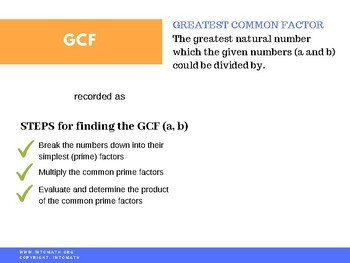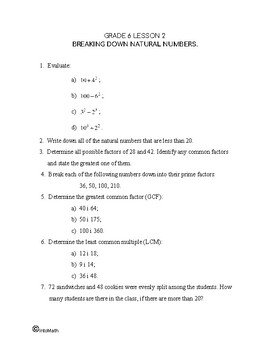CA$2.00 Numbers can be broken down into smaller numbers or themselves and 1. In this lesson we are learning about prime factors, how to determine them and why we need them. Prime factors are numbers that cannot be split further into other whole numbers, other than themselves and 1. Factors in general are numbers that the given number can be divided by. Or whole numbers that are multiplied together to produce another number. The original numbers are factors of the product. If a x b = c then a and b are factors of c. For example, the factors of 8 are 1, 8, 2, 4. In this lesson we are also discussing the concept of the Greatest Common Factor for the given two numbers. The GCF is the largest number the two given numbers could be divided by. The Least Common Multiple is the smallest number that could be divided by the given two numbers.Grade 6 Workbook (16 pages with answers) CA$4.00

For example, the LCM of 4 and 8 is 8, because it could be divided by both 4 and 8 and it is the lowest possible value.

LCM will later be used to determine the Lowest Common Denominator of the two fractions.

Its extremely important to understand the concepts studies in this lesson and learn all of the different methods of breaking down natural numbers. These concepts are the foundation for some of the more complex concepts.